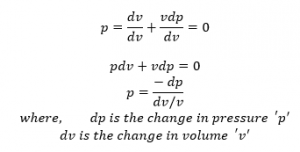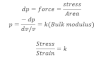# Velocity Of Sound In Gas

Newton’s formula for the velocity of sound in the gas medium is given by,
μ = √K/S
Where k is bulk modulus of medium (gas air) and s be the density of medium.
According to the newton’s sound waves travels through the air of gas. Isothermally i.e. the vibration of the temperature in the region of compression and rarefaction are negligible or an isothermal process Boyle’s law holds true.

i.e. pv = constant

Differentiating with respect to v,Due to the propagation of sound wave.
Here, dv/v represents the volumetric strain and dp represents the force applied by wave per unit area of medium.Therefore the velocity of sound on the air or gas.
μ = √P/S

This is the Newton’s formula for the velocity of sound in air (or gas).

At NTP,
P = 1.01 × 105 N/M2
S = 1.29 Kg/m3
μ = 1.01 × 105 / (1.29) = 281 m/s

Experimentally, velocity of the sound in the air is about 332ms at NTP. This means the velocity of the sound given by Newton’s formula did not agree with experimental value. Therefore, there must be something wrong in Newton’s formula which is called by Laplace.